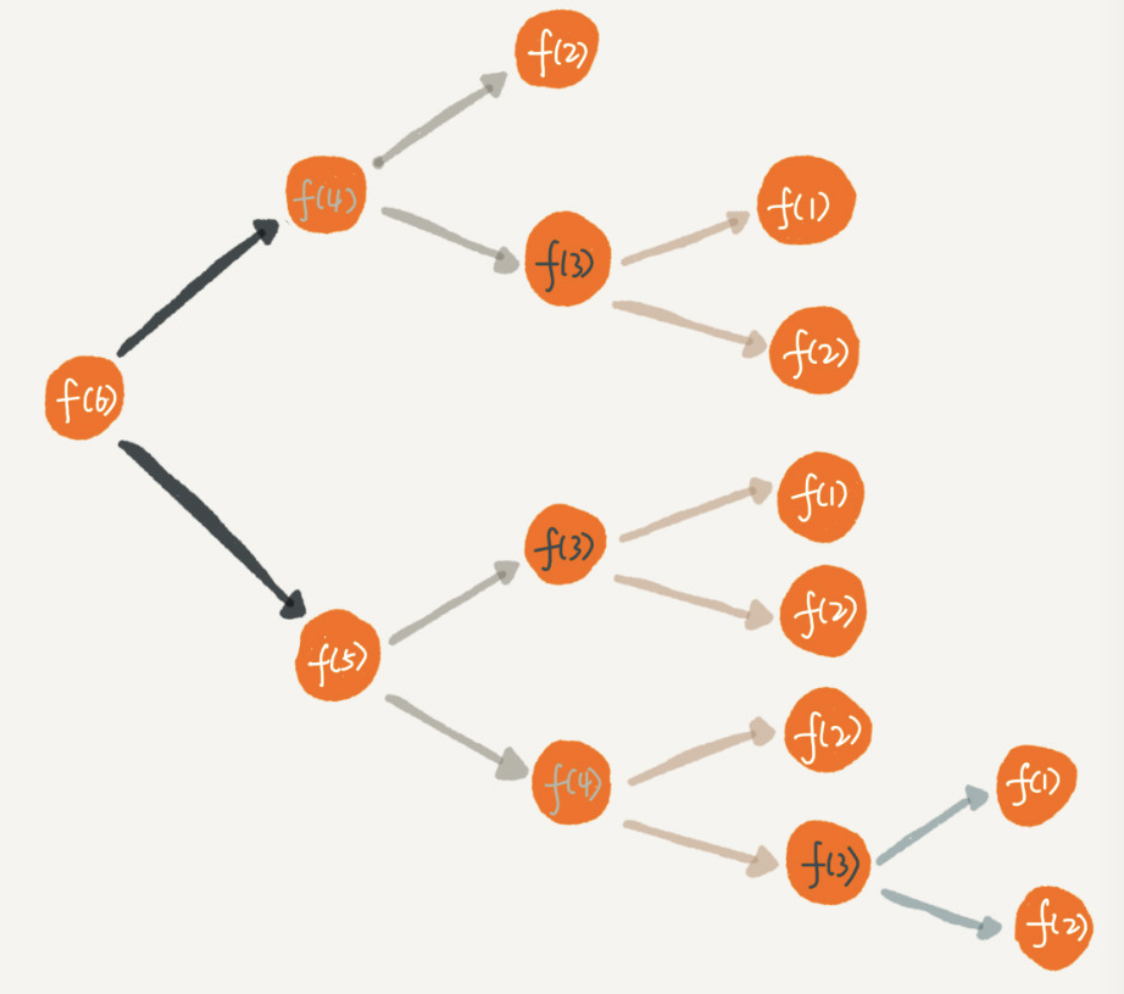# 如何解决递归重复计算问题

在日常编写递归函数的时候，存在一个问题，即重复计算（如下图所示）。解决该问题的常用方法之一是将已经计算过的节点值用一个map容器保存起来，每次递归先查找该容器中是否存在该节点(key)，如果存在则直接返回对应的值(value)；否则计算，并将新的节点(key)和值(value)存入map中，具体参考实现代码以及注释，比较清楚C++实现：

#include<iostream>
#include<map>
using namespace std;

//用于存放递归计算的中间值
map<int,int> m;

int f(int n){
if(n==1) return 1;
if(n==2) return 2;

if(m.count(n)){
//cout<<1<<endl;  //显示节省了多少次递归
//查找map，如果已经计算过则直接返回该值，不再重复计算
return m[n];
}

int res=f(n-1)+f(n-2);
m.insert(pair<int,int> (n,res)); //把新的中间值存入map
return res;
}

int main(){
int n;
cin>>n;
cout<<f(n)<<endl;
}